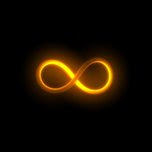## Monday, June 7, 2010

### INFINITY OF FUNThis month I thought we'd have some fun with math.
And what's more fun than infinity? Nothing! Get it? You know infinity—being nothing (I can hear you gentle readers groaning.) . . . oh never mind. Anyway, this is Part I of II where we discuss the fun (and headaches) mathematicians, scientists, and philosophers have with infinity.

If you leave this article remembering only one thing let it be that infinity is NOT a number—it's an idea—and as such it can't be used in algebraic operations. To demonstrate, I will prove that 2 = 1.

1. A = B (There is some A that equals some B. This is a given)
2. A + A = B + A (Adding A to both sides)
3. 2A = B + A (Grouping)
4. 2A - 2B = B + A - 2B (Subtracting 2B from both sides)
5. 2(A - B) = B +A - 2B (Factoring left hand side)
6. 2(A - B) = 1(A - B) (Simplifying right hand side)
7. 2 = 1 (Cancel (A-B) from both sides)

The above are all traditional algebraic operations—however, I tortured them into confessing to an absurd conclusion. One of the steps above is forbidden. Do you know which? I went from step (6) to (7) by dividing both sides by (A - B).

You might say: So?

Well since you asked. Recall line (1) states A = B, meaning that (A - B) = 0. And, as your middle school teacher taught you, dividing by zero is a no no. The reason being (if you recall my lame opening joke) there is an infinite number of NOTHING(s) in EVERYTHING. Therefore dividing anything by 0 is infinity. And infinity is not a number.

Since infinity doesn't make any mathematical sense, scientists have a cheat called "taking the limit" to solve these division by zero issues. In other words, taking a limit of an equation means the variable in question may approach infinity, but must always fall short. Consider it a stop sign. Here is an example where I show you how to use a "limit" to avoid the "division by zero" problem AND I'll do this while using clichés to confirm: The less you know the more money you make.

Cliché 1: Knowledge is Power.
Cliché 2: Time is Money.

1. Power = Work / Time (Physics formula)
2. Knowledge = Power (Restating Clichés)
Time = Money
3. Knowledge = Work / Money (Combining (1) & (2) using substitution)
4. Money = Work / Knowledge (Solving (3) for Money)

So as you can see from (4)—the moral of this proof—as the Limit of Knowledge approaches zero, Money approaches infinity, regardless of the amount of Work done.

In other words by taking the downward "limit," knowledge (the denominator) never quite reaches zero and the amount of Money is capped, never reaching infinity.

Before leaving, here are some parting questions to consider. I might even give the answer in a future post.

Does infinity / infinity = 1?
Does infinity - infinity = 0?

I'll see you next month for Part II of our discussion on infinity. Here's a preview: I get shot (why would anyone want to do that?) by a gun, but the bullet never reaches me. I will be saved by math!

by David Siegel Bernstein